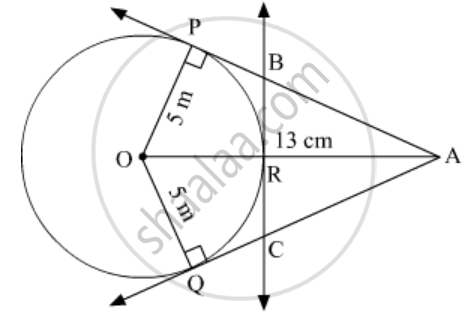Advertisement Remove all ads

# A is a Point at a Distance 13 Cm from the Centre O of a Circle of Radius 5 Cm . Ap and Aq Are the Tangents to the Circle at P and Q . If a Tangent Bc is Drawn at a Point R Lying on the Minor Arc Pq - Mathematics

Short Note

is a point at a distance 13 cm from the centre O of a circle  of radius  5 cm . AP and AQ  are the tangents to the circle at P and Q . If a tangent BC is drawn at a point R lying on the minor arc PQ to intersect  AP at  and AQ at C , find the perimeter of the $∆$ABC .

Advertisement Remove all ads

#### SolutionA is a point 13 cm from centre O. AP and AQ are the tangents to the circle with centre O.
AP = AQ
In ∆APO,

${AP}^2 + {OP}^2 = {AO}^2$

$\Rightarrow {AP}^2 = {AO}^2 - {OP}^2$

$\Rightarrow {AP}^2 = {13}^2 - 5^2$

$\Rightarrow {AP}^2 = 169 - 25 = 144$

$\Rightarrow AP = 12 cm$

Now In ∆ABC,
Perimeter = AB + BC + AC
= AB + BR + RC + AC
= AB + BP + CQ + AC           ( BR = BP, RC = CQ)
=  AP + AQ
= 12 + 12
= 24 cm

Is there an error in this question or solution?
Advertisement Remove all ads

#### APPEARS IN

RD Sharma Class 10 Maths
Chapter 8 Circles
Exercise 8.2 | Q 24 | Page 35
Advertisement Remove all ads
Advertisement Remove all ads
Share
Notifications

View all notifications

Forgot password?